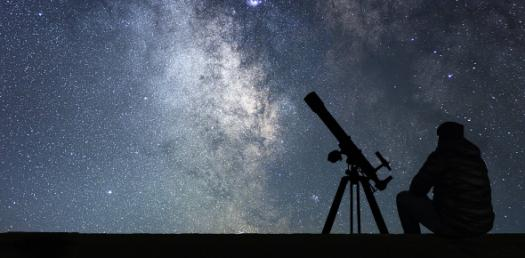# Quiz: Are You Ready To Take A Short Astronomy Test?

12 Questions | Attempts: 359
ShareSettings.

• 1.
If an object's velocity is doubled, its momentum is
• A.

Halved

• B.

Unchanged

• C.

Doubled

• D.

• E.

Dependent on its acceleration.

• 2.
Suppose an object is moving in a straight line at 50 mi/hr. According to Newton's first law of motion, the object will
• A.

Continue to move in the same way forever, no matter what happens.

• B.

Continue to move in the same way until it is acted upon by a force.

• C.

Eventually slow down and come to a stop.

• D.

Continue to move in a straight line forever if it is in space, but fall to the ground if it is on Earth.

• 3.
How does the Space Shuttle take off?
• A.

Its rocket engines push against the launch pad, propelling the shuttle upwards.

• B.

By converting mass-energy to kinetic energy

• C.

By achieving lift from its wings in the same way that airplanes do

• D.

Hot gas shoots out from the rocket and, by conservation of momentum, the shuttle moves in the opposite direction.

• E.

The hot rocket exhaust expands the air beneath the shuttle, propelling it forward.

• 4.
The movement of a pool ball, after being struck by a cue, is an example of
• A.

Newton's first law of motion.

• B.

Newton's second law of motion.

• C.

Newton's third law of motion.

• D.

The universal law of gravitation.

• E.

Conservation of momentum.

• 5.
What quantities does angular momentum depend upon?
• A.

Mass and velocity

• B.

• C.

• D.

• E.

Momentum and angular velocity

• 6.
Considering Einstein's famous equation, E = mc2, which of the following statements is true?
• A.

Mass can be turned into energy, but energy cannot be turned back into mass.

• B.

It takes a large amount of mass to produce a small amount of energy.

• C.

A small amount of mass can be turned into a large amount of energy.

• D.

You can make mass into energy if you can accelerate the mass to the speed of light.

• E.

One kilogram of mass represents 1 joule of energy.

• 7.
Which of the following statements correctly describes the law of conservation of energy?
• A.

An object always has the same amount of energy.

• B.

Energy can change between many different forms, such as potential, kinetic, and thermal, but it is ultimately destroyed.

• C.

The total quantity of energy in the universe never changes.

• D.

The fact that you can fuse hydrogen into helium to produce energy means that helium can be turned into hydrogen to produce energy.

• E.

It is not really possible for an object to gain or lose potential energy, because energy cannot be destroyed.

• 8.
Where does the energy come from that your body uses to keep you alive?
• A.

It is produced from the radiative energy of the Sun on your skin.

• B.

It comes from the foods you eat.

• C.

It comes from the water you drink.

• D.

It is in the air that you breathe.

• E.

It is created during the time that you rest or sleep.

• 9.
According to the universal law of gravitation, the force due to gravity is
• A.

Directly proportional to the square of the distance between objects.

• B.

Inversely proportional to the square of the distance between objects.

• C.

Directly proportional to the distance between objects.

• D.

Inversely proportional to the distance between objects.

• E.

Not dependent on the distance between objects.

• 10.
According to the universal law of gravitation, if you double the masses of both attracting objects, then the gravitational force between them will
• A.

Not change at all.

• B.

Increase by a factor of 2.

• C.

Decrease by a factor of 2.

• D.

Increase by a factor of 4.

• E.

Decrease by a factor of 4.

• 11.
The allowed shapes for orbits under the force of gravity are
• A.

Ellipses only.

• B.

Ellipses and spirals.

• C.

Ellipses, parabolas, and hyperbolas.

• D.

Ellipses, spirals, and parabolas.

• E.

Spirals, circles, and squares.

• 12.
At which lunar phase(s) are tides least pronounced (e.g., the lowest high tides)?
• A.

First quarter

• B.

New moon

• C.

Full moon

• D.

Both new and full moons

• E.

Both first and third quarters

## Related TopicsBack to top
×

Wait!
Here's an interesting quiz for you.